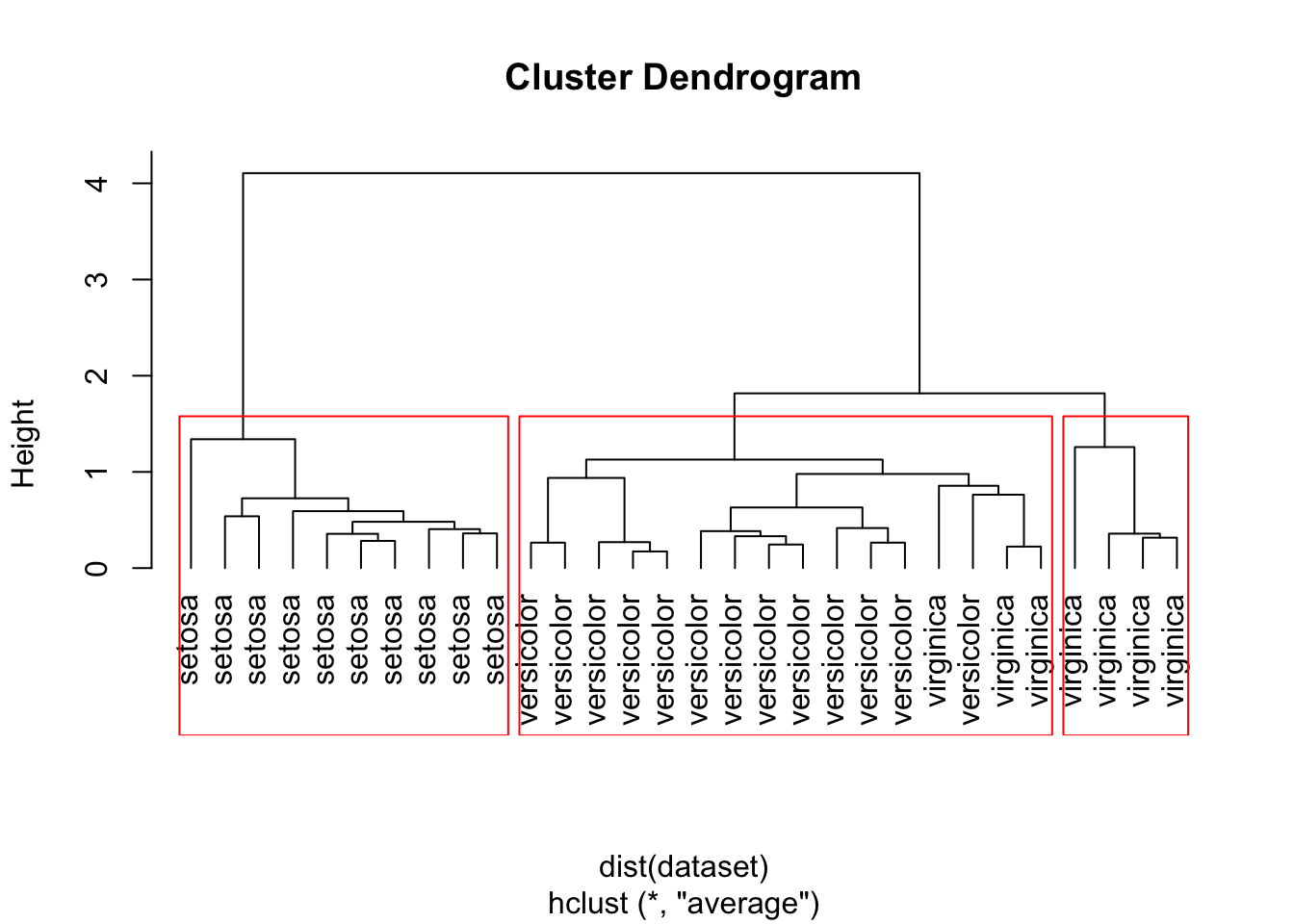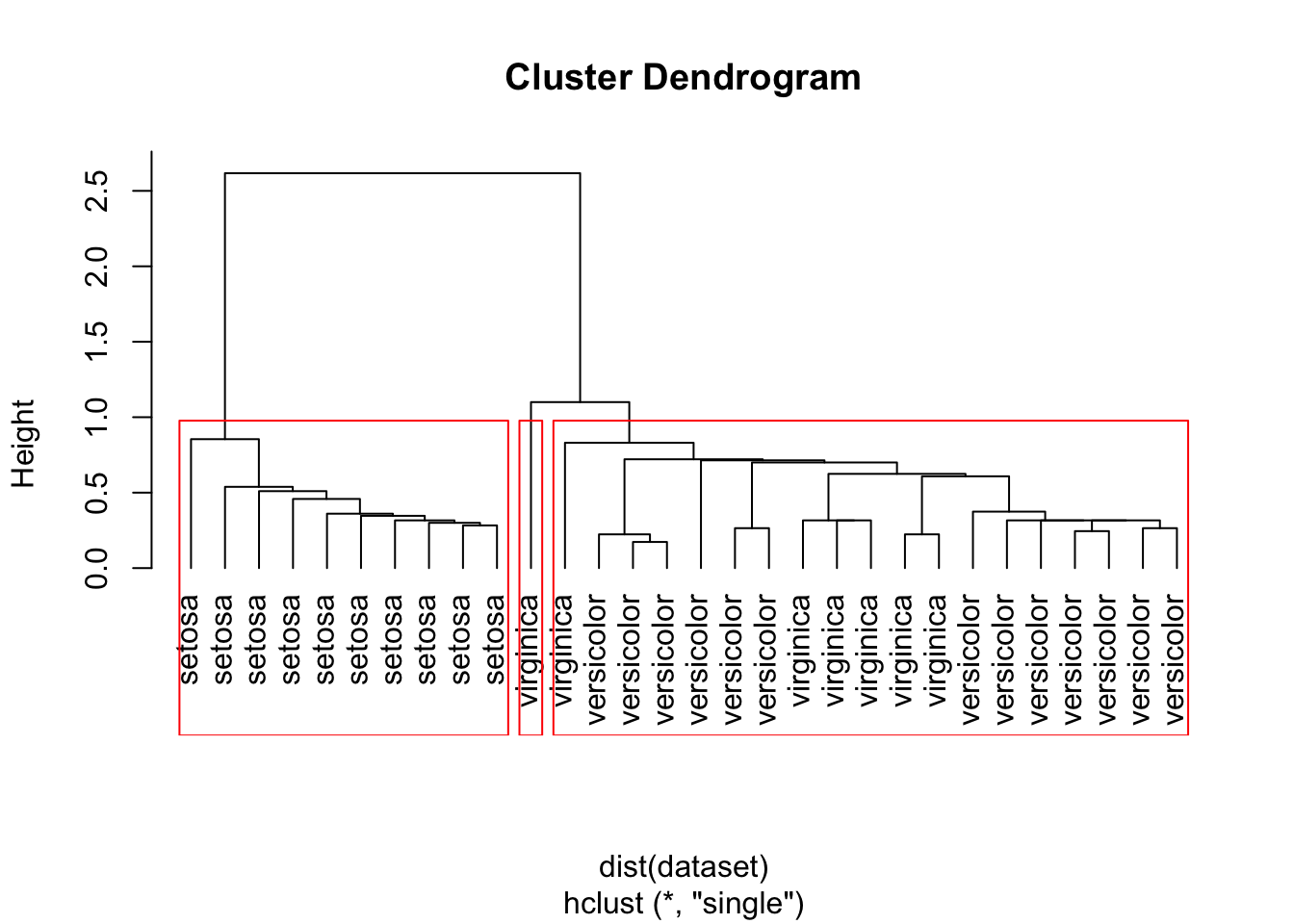## Hierarchical Clustering

For hierarchical clustering we will use the iris dataset. To make the clustering dendrogram less crowded we will select 30 instances from the iris dataset.

indices <- sample(1:nrow(iris), 30)
dataset <- iris[indices, -5]

To do hierachical clustering we will use the hclust method:

hc <- hclust(dist(dataset), method="ave")

We have different options to use in the method argument to define the distance between the clusters as:

• the distance between the closests points of the clusters (single)
• the distance between the farthests points of the clusters (complete)
• the average distance between all points in the clusters (average)
• the distance between the centroids of the clusters (centroid)
• and others (see ?hclust for a list)

To plot the dendrogram and cut the tree into three clusters:

# Plot the dendrogram
plot(hc, hang = -1, labels=iris$Species[indices]) # cut tree into 3 clusters rect.hclust(hc, k=3)# groups contain the distribution of the samples to the clusters/groups groups <- cutree(hc, k=3) ## Using single hc <- hclust(dist(dataset), method="single") # Plot the dendrogram plot(hc, hang = -1, labels=iris$Species[indices])
# cut tree into 3 clusters
rect.hclust(hc, k=3)# groups contain the distribution of the samples to the clusters/groups
groups <- cutree(hc, k=3)